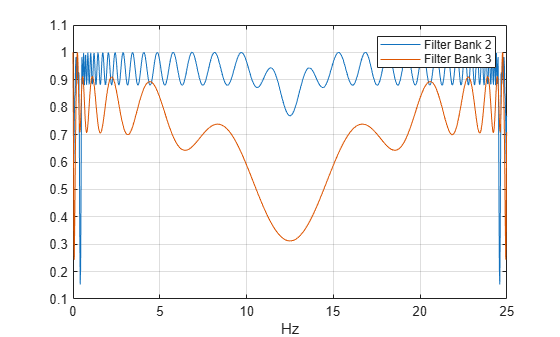# littlewoodPaleySum

Littlewood-Paley sum

## Syntax

``lpsum = littlewoodPaleySum(sf)``
``lpsum = littlewoodPaleySum(sf,fb)``
``[lpsum,f] = littlewoodPaleySum(___)``

## Description

````lpsum = littlewoodPaleySum(sf)` returns the Littlewood-Paley sum for the scattering filter banks in `sf`, the wavelet time scattering network. `lpsum` is an M-by-L matrix, where M is the number of elements in the Fourier transform of the scattering filters, and L is the number of scattering filter banks. The columns of `lpsum` are ordered by the position of the filter bank in the scattering network. For example, the first column of `lpsum` corresponds to the filter bank used for the first-order scattering coefficients.Since the scattering transform is contractive, the Littlewood-Paley sums will not exceed one.```
````lpsum = littlewoodPaleySum(sf,fb)` returns the Littlewood-Paley sum for the specified filter bank `fb` in `sf`. The argument `fb` is a positive integer between 1 and the number of filter banks in `sf` inclusive. The number of filter banks in `sf` is equal to the number of specified `QualityFactors` in `sf`.```

example

````[lpsum,f] = littlewoodPaleySum(___)` returns the frequencies for the Littlewood-Paley sum. If you specify a sampling frequency in `sf`, `f` is in hertz. If you do not specify a sampling frequency, `f` is in cycles/sample. You can use these output arguments with any of the input syntaxes shown previously.```

## Examples

collapse all

Create a wavelet time scattering network with three filter banks for data sampled at 25 Hz.

```sf = waveletScattering('QualityFactors',[8 4 1],... 'SamplingFrequency',25)```
```sf = waveletScattering with properties: SignalLength: 1024 InvarianceScale: 20.4800 QualityFactors: [8 4 1] Boundary: 'periodic' SamplingFrequency: 25 Precision: 'double' OversamplingFactor: 0 OptimizePath: 0 ```

Plot the Littlewood-Paley sums for the second and third filter banks. Note that the sums do not exceed 1. This shows the filters have been normalized so that the scattering transform is contractive.

```[lpsum,f] = littlewoodPaleySum(sf); plot(f,lpsum(:,2:3)) grid on legend('Filter Bank 2','Filter Bank 3') xlabel('Hz')```## Input Arguments

collapse all

Wavelet time scattering network, specified as a `waveletScattering` object.

Filter bank index in the wavelet time scattering network, specified as a positive integer between 1 and the number of filter banks in `sf` inclusive. The number of filter banks in `sf` is equal to the number of specified `QualityFactors` in `sf`.

Data Types: `double`

## Output Arguments

collapse all

Littlewood-Paley sum for the filter banks in the scattering network `sf`, returned as a real-valued matrix. `lpsum` is an M-by-L matrix, where M is the number of elements in the Fourier transform of the scattering filters and L is the number of scattering filter banks. For example, the first column of `lpsum` corresponds to the filter bank used for the first-order scattering coefficients.

Frequencies for the Littlewood-Paley sum, returned as a real-valued vector. If you specify a sampling frequency in `sf`, `f` is in hertz. If you do not specify a sampling frequency, `f` is in cycles/sample.

Data Types: `double`

## Version History

Introduced in R2018b